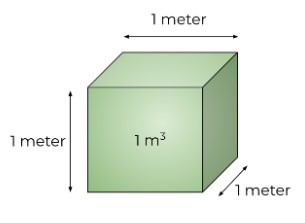# Cubic Meter Calculator

To find cubic meter, fill the required input fields & click calculate button using cubic meter calculator

Formula:
Cubic Meter = Length (m)×Width (m)×Height (m)

Give Us Feedback

## CBM calculator

Cubic meter calculator is a volume computing tool. It finds the volume in cubic meters and several other units.

Furthermore, other units like cubic yards and cubic millimeters are converted to cubic meters in this calculator.

## What is CBM?

CBM stands for Cubic Meters. Just like cubic feet, it is a unit of volume. A cubic meter is a cube with all three lengths of one meter. It is mostly used to measure the volume of consignments.## Cubic meter formula:

Multiply the length, width, and height after the conversion into meters.

Volume in CBM = L (in meters) x W (in meters) x H (in meters)

## How to find the cubic meters?

There are two methods. With the first method, units are changed after the conversion. While using the second method, as the calculator above does, units are changed before the computation.

In the coming examples, you can see the formulas to convert the most used units into meters.

Example:

Find the volume in cubic meters using these measurements.

Height = 300 inches
Width = 6000 millimeters
Length = 2500 centimeters

Solution:

Step 1: Convert all units to meters.

Height:

1 inch = 0.0254 meters
300 inches = 0.0254 x 300
= 7.62 meters

Width:

1 millimeter = 0.001 meters
6000 millimeters = 0.001 x 6000
= 6 meters

Length:

1 centimeter = 0.01 meters
250 centimeters = 0.01 x 250
= 2.5 meters

Step 2: Multiply the lengths.

= L x W x H
= 2.5 x 6 x 7.62
= 114.3 m3

### Math Tools